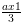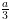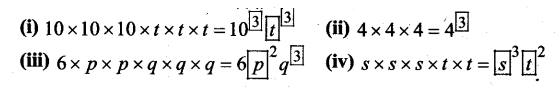• +91 9971497814
• info@interviewmaterial.com

# Chapter 5- बीजगणितीय अवधारणा Ex-5-e Interview Questions Answers

### Related Subjects

Question 1 :
निम्नलिखित गणितीय कथनों पर ध्यान दीजिए तथा अपनी अभ्यास पुस्तिका में बॉक्स के स्थान पर अक्षर संख्याओं के लिए संख्या लिखिए-
(i) 6 + 4 = x
(ii) 3 x 9 = y
(iii) 6 – 2 = a
(iv) b ÷ 2 = 5

(i) 6 + 4 = 10
(ii) 3 x 9 = 27
(iii) 6 – 2 = 4
(iv) 10 ÷ 2 = 5

Question 2 :
ज्ञात कीजिए (ज्ञात करके)-
(i) 10 में से घटाने पर प्राप्त संख्या
(ii) 2x और 3y को जोड़ने पर प्राप्त संख्या
(ii) y की 6 गुनी संख्या
(iv) a में 3 का भाग देने पर प्राप्त संख्या

(i) 10 में से घटाने पर प्राप्त संख्या = 10 – x
(ii) 2x और 3y को जोड़ने पर प्राप्त संख्या = 2x + 3y
(ii) y की 6 गुनी संख्या = y x 6 = 6y
(iv) a में 3 का भाग देने पर प्राप्त संख्या = a ÷ 3 ==Question 3 :
यदि a = 5 तथा b = 9, तो निम्नांकित के मान ज्ञात कीजिए (ज्ञात करके)-
(i) a + 10
(ii) b – 3
(iii) a + b – 14
(iv) a x b
(v) 30 ÷ a

(i) a + 10 = 5 + 10 = 15
(ii) b – 3 = 9 – 3 = 6
(iii) a + b – 14 = 5 + 9 – 14 = 14 – 14 = 0
(iv) a x b = 5 x 9 = 45
(v) 30 ÷ a = 30 ÷ 5 = 6

Question 4 :
विस्तृत रूप को घातांकीय रूप में लिखिए (लिखकर)-
(i) x x x x x x y
(i) q x q x q
(iii) 2 x y x y x y
(iv) 5 x 5 x 5 x x x y x y x y
(v) m x m x m x m

(i) x x x x x x y = x3y
(i) q x q x q = q3
(iii) 2 x y x y x y = 2y3
(iv) 5 x 5 x 5 x x x y x y x y = 125xy3
(v) m x m x m x m = m4

Question 5 : रिक्त स्थानों की पूर्ति अपनी अभ्यास पुस्तिका में कीजिए-

Answer 5 :Question 6 :
अधोलिखित कथनों को देखकर उसमें चर और अचर छाँटिए (अँटकर)
(i) 5x2y2z3
(i) 7x2y2
(iii) m4n2
(iv) a3b5

(i) चर = x, y,z
अचर = 5
(ii)
चर = x, y
अचर = 7
(iii)
चर = m, n
(iv)
चर = a, b

Todays Deals### Chapter 5- बीजगणितीय अवधारणा Ex-5-e Contributorskrishan

Name:
Email:

# Latest News# 9000 interview questions in different categories